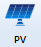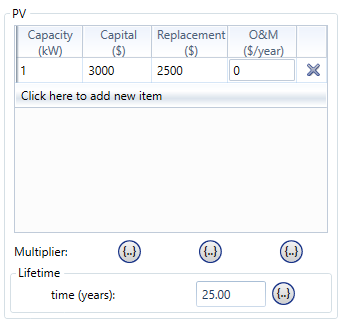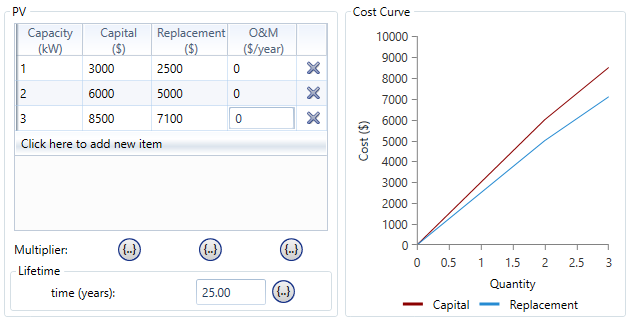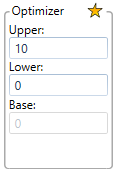# HOMER Pro 3.11

 Navigation: Design > Components Tab Photovoltaic Panels (PV)The PV page allows you to enter the cost, performance characteristics, and orientation of an array of photovoltaic (PV) panels and choose the sizes you want HOMER to consider as it searches for the optimal system. The PV component can represent both flat panel and concentrating PV technologies. You can specify whether a PV is a concentrating PV in the Photovoltaic Library.

Inverter: If the Electrical Bus is set to AC, you specify inverter parameters here.

MPPT: If the Electrical Bus is set to DC, you set the parameters of the maximum power point tracker (DC to DC converter) here.

Temperature: You specify whether to consider the effect of ambient temperature on panel efficiency, and if so set the relevant inputs.

To add a solar component, click the PV button under the Components tab in the toolbar. On the PV Set Up page, select an option from the drop-down list and click the Add PV button. On the PV page, you can manage information for the model.

## Costs

The Costs table includes the initial Capital cost and Replacement cost per kilowatt of the PV system, as well as annual operation and maintenance (O&M) costs per kilowatt.When specifying the capital and replacement costs, be sure to account for all costs associated with the PV system, which may include:

PV panels

Mounting hardware

Tracking system

Wiring

Installation

You can include the costs of the power electronics in the capital cost, or account for them separately in the MPPT or Inverter tab.

Note: The capital cost is the initial purchase price, the replacement cost is the cost of replacing the PV system at the end of its lifetime, and the O&M cost is the annual cost of operating and maintaining the PV system.

## Cost Curve

In the Costs table, enter the PV cost curve, meaning the way the cost varies with size. Typically this requires only a single row because analysts often assume that PV costs vary linearly with size. In the sample above, the capital cost of PV panels is specified at \$3,000/kW and the replacement cost is specified at \$2,500/kW. The operating and maintenance (O&M) cost is specified as 0.

If the cost of the PV subsystem was not linear with size, you can enter multiple rows of data in the Cost table. For example, if the marginal capital and replacement costs dropped to \$2,500/kW and \$2,100/kW, respectively, for quantities above 2 kW, fill in the Cost table as follows:If HOMER then simulates a system with a PV array size of 0.1 kW, it would extrapolate from the 1 kW and 2 kW costs, giving a capital cost of \$300. For a PV array size of 2.5 kW, HOMER would interpolate between the 2 kW costs and the 3 kW costs, giving a capital cost of \$7,250. For a PV array size of 6 kW, HOMER would extrapolate from the 2 kW and 3 kW costs, giving a capital cost of \$16,000.

Note: The capital cost is the initial purchase price, the replacement cost is the cost of replacing the PV panels at the end of their lifetime, and the O&M cost is the annual cost of operating and maintaining the PV array.

## Search Space

Click the MPPT or Inverter tab to see the Search Space. Enter the nominal capacity of the PV in kW, or enter several quantities for HOMER to consider in the system optimization. Include a zero if you wanat HOMER to consider systems without this PV.

Click the Star icon to enable the optimizer. The search space is replaced by a lower bound and an upper bound. With the optimizer turned on, HOMER automatically finds the best capacity for you.See the help article about Optimization for a more detailed explanation of HOMER's optimizer.

## PV Inputs

From the main section of the PV page, you can edit the following inputs.

 Variable Description Electrical Bus This determines whether the PV array produces AC or DC power. All PV cells produce DC electricity, but some PV arrays have built-in inverters to convert to AC. Lifetime The number of years before the PV panels must be replaced at the replacement cost specified in the costs table. Derating Factor A scaling factor applied to the PV array power output to account for reduced output in real-world operating conditions compared to operating conditions at which the array was rated.

Note: To the right of each numerical input is a sensitivity button () that allows you to do a sensitivity analysis on that variable. For more information, see Why Would I Do a Sensitivity Analysis?

## Inverter

If the PV system is on the AC electrical bus, you can specify a dedicated inverter. You can define the Cost table, size (search space), and lifetime in a similar manner as other components. You can also specify the efficiency with a single value, or check "Use efficiency table," and enter values for efficiency versus input load percentage.

If you do not want to model the inverter, check the "Ignore dedicated converter" box.

## MPPT

A Maximum Power Point Tracker (or MPPT) is a DC to DC converter that matches the PV to the DC bus voltage, while varying the voltage of the PV array itself to maximize the power output. The inputs for the MPPT are identical to those for the inverter, described above.

The Advanced Input tab contains options that affect the calculation of the PV power output. The article How HOMER Calculates the Radiation Incident on the PV contains more information on ground reflectance, panel slope, and panel azimuth.

 Variable Description Ground Reflectance The fraction of solar radiation incident on the ground that is reflected, in % Tracking System The type of tracking system used to direct the PV panels towards the sun Use default slope If this input is checked, the slope input is disabled and the slope is set to match the latitude Panel Slope The angle at which the panels are mounted relative to horizontal, in degrees Use default azimuth If this input is checked, the azimuth input is disabled and the azimuth is set to 0 or 180 degrees for projects in the northern or southern hemisphere, respectively Panel Azimuth The direction towards which the panels face, in degrees

## Temperature

The Temperature tab allows you to set or ignore temperature effects. See How HOMER Calculates the PV Array Power Output for detailed information on temperature effects on power, nominal operating cell temperature, and efficiency at standard test conditions.

 Variable Description Consider Effect of Temperature Whether HOMER considers the effect of PV cell temperature on the power output of the PV array Temperature Coefficient of Power A number indicating how strongly the power output of the PV array depends on cell temperature, in %/degrees Celsius Nominal Operating Cell Temperature The cell temperature at 0.8 kW/m2 and 20°C ambient temperature in degrees Celsius Efficiency at Standard Test Conditions The maximum power point efficiency under standard test conditions, in %